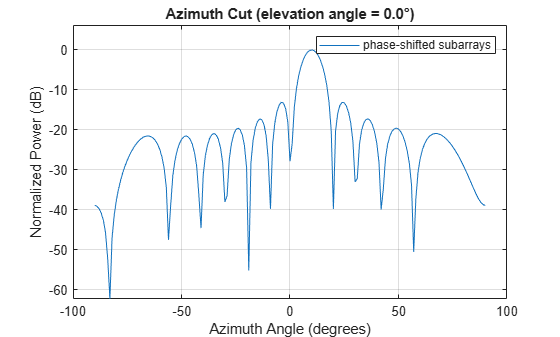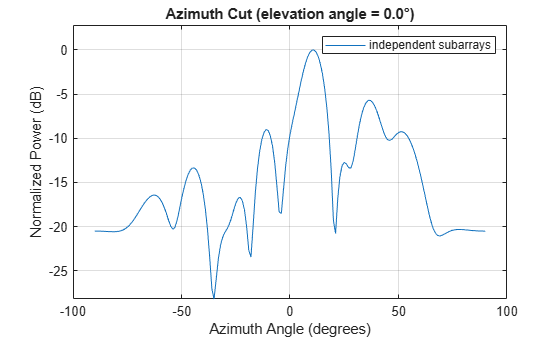# step

System object: phased.ReplicatedSubarray
Package: phased

Output responses of subarrays

## Syntax

``RESP = step(H,FREQ,ANG,V)``
``RESP = step(H,FREQ,ANG,V,STEERANGLE)``
``RESP = step(H,FREQ,ANG,V,WS)``

## Description

Note

Starting in R2016b, instead of using the `step` method to perform the operation defined by the System object™, you can call the object with arguments, as if it were a function. For example, ```y = step(obj,x)``` and `y = obj(x)` perform equivalent operations.

example

````RESP = step(H,FREQ,ANG,V)` returns the responses, `RESP`, of the subarrays in the array, at operating frequencies specified in `FREQ` and directions specified in `ANG`. `V` is the propagation speed. The elements within each subarray are connected to the subarray phase center using an equal-path feed.```
````RESP = step(H,FREQ,ANG,V,STEERANGLE)` uses `STEERANGLE` as the steering direction of the subarray. This syntax is available when you set the `SubarraySteering` property to either `'Phase'` or `'Time'`.```

example

````RESP = step(H,FREQ,ANG,V,WS)` uses `WS` as the subarray element weights. This syntax is available when you set the `SubarraySteering` property to `'Custom'`.```

Note

The object performs an initialization the first time the object is executed. This initialization locks nontunable properties and input specifications, such as dimensions, complexity, and data type of the input data. If you change a nontunable property or an input specification, the System object issues an error. To change nontunable properties or inputs, you must first call the `release` method to unlock the object.

## Input Arguments

`H`

Phased array formed by replicated subarrays.

`FREQ`

Operating frequencies of array in hertz. `FREQ` is a row vector of length L. Typical values are within the range specified by a property of `H.Subarray.Element`. That property is named `FrequencyRange` or `FrequencyVector`, depending on the type of element in the array. The element has zero response at frequencies outside that range.

`ANG`

Directions in degrees. `ANG` can be either a 2-by-M matrix or a row vector of length M.

If `ANG` is a 2-by-M matrix, each column of the matrix specifies the direction in the form [azimuth; elevation]. The azimuth angle must be between –180 and 180 degrees, inclusive. The elevation angle must be between –90 and 90 degrees, inclusive.

If `ANG` is a row vector of length M, each element specifies a direction’s azimuth angle. In this case, the corresponding elevation angle is assumed to be 0.

`V`

Propagation speed in meters per second. This value must be a scalar.

`STEERANGLE`

Subarray steering direction. `STEERANGLE` can be either a 2-element column vector or a scalar.

If this argument is a 2-element column vector, it has the form [azimuth; elevation]. The azimuth angle must be between –180° and 180°, inclusive. The elevation angle must be between –90° and 90°, inclusive.

If `STEERANGLE` is a scalar, it specifies the direction’s azimuth angle. In this case, the elevation angle is assumed to be 0°.

#### Dependencies

To enable this argument, set the `SubarraySteering` to `'Phase'` or `'Time'`.

`WS`

Subarray element weights

Subarray element weights, specified as complex-valued NSE-by-N matrix. Weights are applied to the individual elements within a subarray. All subarrays have the same dimensions and sizes. NSE is the number of elements in each subarray and N is the number of subarrays. Each column of the matrix specifies the weights for the corresponding subarray.

#### Dependencies

To enable this argument, set the `SubarraySteering` to `'Custom'`.

## Output Arguments

 `RESP` Voltage responses of the subarrays of the phased array. The output depends on whether the array supports polarization or not. If the array is not capable of supporting polarization, the voltage response, `RESP`, has the dimensions N-by-M-by-L. The first dimension, N , represents the number of subarrays in the phased array, the second dimension, M, represents the number of angles specified in `ANG`, while L represents the number of frequencies specified in `FREQ`. Each column of `RESP` contains the responses of the subarrays for the corresponding direction specified in `ANG`. Each of the L pages of `RESP` contains the responses of the subarrays for the corresponding frequency specified in `FREQ`.If the array is capable of supporting polarization, the voltage response, `RESP`, is a MATLAB® `struct` containing two fields, `RESP.H` and `RESP.V`, each having dimensions N-by-M-by-L. The field, `RESP.H`, represents the array’s horizontal polarization response, while `RESP.V` represents the array’s vertical polarization response. The first dimension, N , represents the number of subarrays in the phased array, the second dimension, M, represents the number of angles specified in `ANG`, while L represents the number of frequencies specified in `FREQ`. Each of the M columns contains the responses of the subarrays for the corresponding direction specified in `ANG`. Each of the L pages contains the responses of the subarrays for the corresponding frequency specified in `FREQ`.

## Examples

expand all

Calculate the response at boresight for two 2-element ULA arrays that form subarrays of a 4-element ULA array of short-dipole antenna elements.

Create a two-element ULA of short-dipole antenna elements. Then, arrange two copies to form a 4-element ULA.

```antenna = phased.ShortDipoleAntennaElement; array = phased.ULA('Element',antenna,'NumElements',2,'ElementSpacing',0.5); replicatedarray = phased.ReplicatedSubarray('Subarray',array,... 'Layout','Rectangular','GridSize',[1 2],... 'GridSpacing','Auto');```

Find the response of each subarray at boresight. Assume the operating frequency is 1 GHz and the wave propagation speed is the speed of light.

```c = physconst('LightSpeed'); resp = replicatedarray(1.0e9,[0;0],c)```
```resp = struct with fields: H: [2x1 double] V: [2x1 double] ```

Create an array consisting of three copies of a 4-element ULA having elements spaced 1/2 wavelength apart. The array operates at 300 MHz.

```c = physconst('LightSpeed'); fc = 300e6; lambda = c/fc; subarray = phased.ULA(4,0.5*lambda);```

Steer all subarrays by a common phase shift to 10 degrees azimuth.

```array = phased.ReplicatedSubarray('Subarray',subarray,'GridSize',[1 3], ... 'SubarraySteering','Phase','PhaseShifterFrequency',fc); steer_ang = [10;0]; sv_array = phased.SteeringVector('SensorArray',array,... 'PropagationSpeed',c); wts_array = sv_array(fc,steer_ang); pattern(array,fc,-90:90,0,'CoordinateSystem','Rectangular',... 'Type','powerdb','PropagationSpeed',c,'Weights',wts_array,... 'SteerAngle',steer_ang); legend('phase-shifted subarrays')```Compute independent subarray weights from subarray steering vectors. The weights point to 5, 15, and 30 degrees azimuth. Set the `SubarraySteering` property to `'Custom'` .

```steer_ang_subarrays = [5 15 30;0 0 0]; sv_subarray = phased.SteeringVector('SensorArray',subarray,... 'PropagationSpeed',c); wc = sv_subarray(fc,steer_ang_subarrays); array.SubarraySteering = 'Custom'; pattern(array,fc,-90:90,0,'CoordinateSystem','Rectangular',... 'Type','powerdb','PropagationSpeed',c,'Weights',wts_array,... 'ElementWeight',conj(wc)); legend('independent subarrays') hold off```